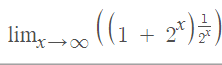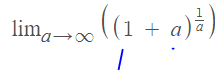# Calculate the limit

LCSphysicist
Homework Statement:
.
Relevant Equations:
(1 + a^x)^(a^(-x))
(1 + a^x)^(a^(-x))
Let's assume a, say, two.

the limit of it, with x tending to infinity,is one, but i was thinking...
Calling 2^x by a, we have that when x tend to infinity, so do a, So:that is euler number no? Contradictory... where am i wrong?

#### Attachments

Eclair_de_XII
one

That's what I got. The way I usually deal with limits of this form is to express it as a function of the exponential function and the natural logarithm, then use l'Hopital's rule on the power.

LCSphysicist
That's what I got. The way I usually deal with limits of this form is to express it as a function of the exponential function and the natural logarithm, then use l'Hopital's rule on the power.
Yes but, what is wrong in the second case?

Eclair_de_XII
I think that the problem is that ##a## is not bounded, and it would not be the same as the limit for Euler's number if you tried to put it that way. For example, if you let ##a=\frac{1}{n^2}##, then as ##a\rightarrow \infty##, ##n^2\rightarrow 0^+##. Then your second limit becomes:

##\lim_{a \rightarrow \infty}(1+a)^{\frac{1}{a}}=\lim_{n^2\rightarrow 0}(1+\frac{1}{n^2})^{n^2}\neq e##

Maybe if you switched around the negative signs in the equation in the Relevant Equations part of your post, it would be some function of Euler's number.

•LCSphysicist
Homework Helper
Gold Member
Euler's number is ##\lim (1+\frac{1}{n})^n ## as ##n\to \infty## or equivalently ##\lim (1+n)^{\frac{1}{n}}## as ##n\to 0##.

BUT you have ##\lim (1+n)^{\frac{1}{n}}## as ##n\to \infty##

••SammyS, FactChecker and LCSphysicist
LCSphysicist
Euler's number is ##\lim (1+\frac{1}{n})^n ## as ##n\to \infty## or equivalently ##\lim (1+n)^{\frac{1}{n}}## as ##n\to 0##.

BUT you have ##\lim (1+n)^{\frac{1}{n}}## as ##n\to \infty##
Oh so i was wrong to think that the third therm was too equivalent.
I really messed me up, the terms between the parentheses need to tend to one, not to infinity... thank you both guys

•Delta2